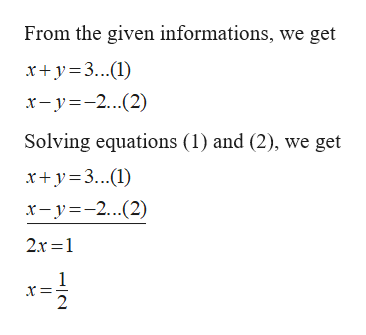# Two numbers total 3. Their difference is -2. Find the two numbers?

Question

Two numbers total 3. Their difference is -2. Find the two numbers?

check_circleExpert Solution
Step 1

Given:

Sum of two numbers is 3 and their difference is -2.

Step 2

Calculation:

Let x and y be the two num...help_outlineImage TranscriptioncloseFrom the given informations, we get xy3.) x-y-2(2) Solving equations (1) and (2), we get xy3() x-y2(2) 2r 1 2 fullscreen

### Want to see the full answer?

See Solution

#### Want to see this answer and more?

Solutions are written by subject experts who are available 24/7. Questions are typically answered within 1 hour*

See Solution
*Response times may vary by subject and question
Tagged in

### Algebra